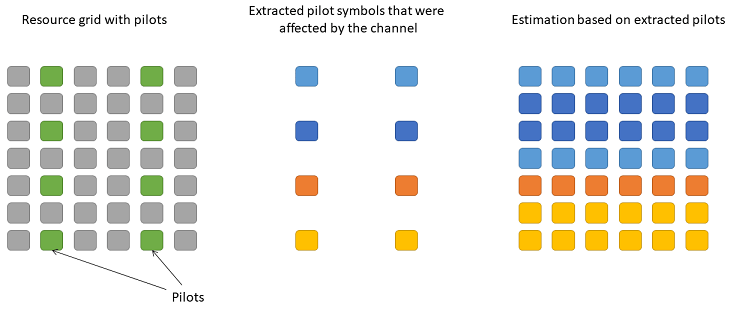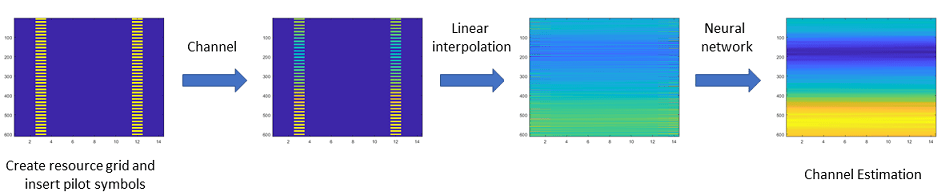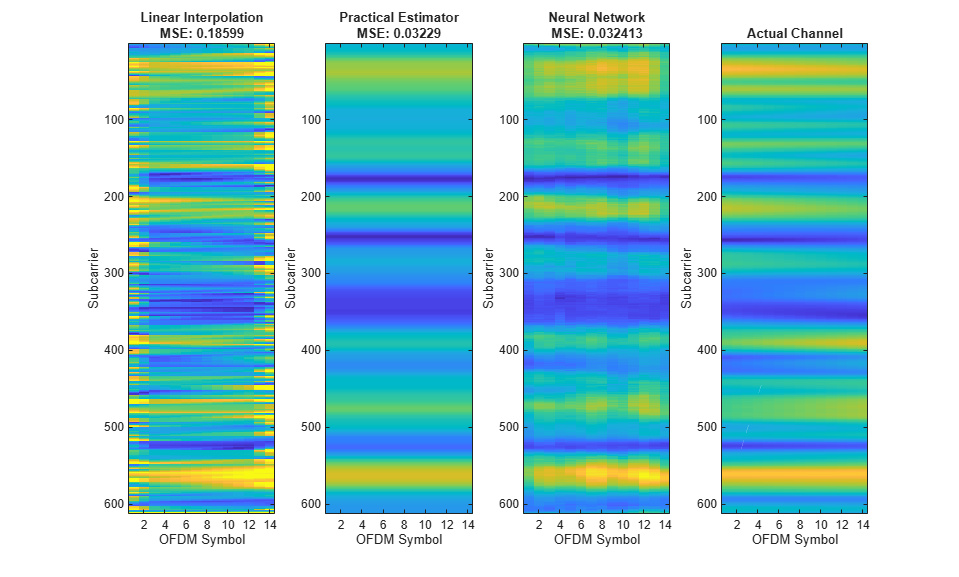# Deep Learning Data Synthesis for 5G Channel Estimation

This example shows how to train a convolutional neural network (CNN) for channel estimation using Deep Learning Toolbox™ and data generated with 5G Toolbox™. Using the trained CNN, you perform channel estimation in single-input single-output (SISO) mode, utilizing the physical downlink shared channel (PDSCH) demodulation reference signal (DM-RS).

### Introduction

The general approach to channel estimation is to insert known reference pilot symbols into the transmission and then interpolate the rest of the channel response by using these pilot symbols.For an example showing how to use this channel estimation approach, see NR PDSCH Throughput.

You can also use deep learning techniques to perform channel estimation. For example, by viewing the PDSCH resource grid as a 2-D image, you can turn the problem of channel estimation into an image processing problem, similar to denoising or super-resolution, where CNNs are effective.

Using 5G Toolbox, you can customize and generate standard-compliant waveforms and channel models to use as training data. Using Deep Learning Toolbox, you can use this training data to train a channel estimation CNN. This example shows how to generate such training data and how to train a channel estimation CNN. The example also shows how to use the channel estimation CNN to process images that contain linearly interpolated received pilot symbols. The example concludes by visualizing the results of the neural network channel estimator in comparison to practical and perfect estimators.### Neural Network Training

Neural network training consists of these steps:

• Data generation

• Splitting the generated data into training and validation sets

• Defining the CNN architecture

• Specifying the training options, optimizer, and learning rate

• Training the network

Due to the large number of signals and possible scenarios, training can take several minutes. By default, training is disabled, a pretrained model is used. You can enable training by setting `trainModel` to true.

```trainModel = false; ```

If you have Parallel Computing Toolbox™ installed and a supported CUDA-enabled NVIDIA® GPU set up, the network training uses GPU acceleration by default. The `trainNetwork` (Deep Learning Toolbox) function allows you to override this default behaviour. For a list of supported GPUs, see GPU Support by Release (Parallel Computing Toolbox).

Data generation is set to produce 256 training examples or training data sets. This amount of data is sufficient to train a functional channel estimation network on a CPU in a reasonable time. For comparison, the pretrained model is based on 16,384 training examples.

Training data of the CNN model has a fixed size dimensionality, the network can only accept 612-by-14-by-1 grids, i.e. 612 subcarriers, 14 OFDM symbols and 1 antenna. Therefore, the model can only operate on a fixed bandwidth allocation, cyclic prefix length, and a single receive antenna.

The CNN treats the resource grids as 2-D images, hence each element of the grid must be a real number. In a channel estimation scenario, the resource grids have complex data. Therefore, the real and imaginary parts of these grids are input separately to the CNN. In this example, the training data is converted from a complex 612-by-14 matrix into a real-valued 612-by-14-by-2 matrix, where the third dimension denotes the real and imaginary components. Because you have to input the real and imaginary grids into the neural network separately when making predictions, the example converts the training data into 4-D arrays of the form 612-by-14-by-1-by-2N, where N is the number of training examples.

To ensure that the CNN does not overfit the training data, the training data is split into validation and training sets. The validation data is used for monitoring the performance of the trained neural network at regular intervals, as defined by `valFrequency`, approximately 5 per epoch. Stop training when the validation loss stops improving. In this instance, the validation data size is the same as the size of a single mini-batch due to the small size of the data set.

The returned channel estimation CNN is trained on various channel configurations based on different delay spreads, doppler shifts, and SNR ranges between 0 and 10 dB.

```% Set the random seed for reproducibility (this has no effect if a GPU is % used) rng(42) if trainModel % Generate the training data [trainData,trainLabels] = hGenerateTrainingData(256); % Set the number of examples per mini-batch batchSize = 32; % Split real and imaginary grids into 2 image sets, then concatenate trainData = cat(4,trainData(:,:,1,:),trainData(:,:,2,:)); trainLabels = cat(4,trainLabels(:,:,1,:),trainLabels(:,:,2,:)); % Split into training and validation sets valData = trainData(:,:,:,1:batchSize); valLabels = trainLabels(:,:,:,1:batchSize); trainData = trainData(:,:,:,batchSize+1:end); trainLabels = trainLabels(:,:,:,batchSize+1:end); % Validate roughly 5 times every epoch valFrequency = round(size(trainData,4)/batchSize/5); % Define the CNN structure layers = [ ... imageInputLayer([612 14 1],'Normalization','none') convolution2dLayer(9,64,'Padding',4) reluLayer convolution2dLayer(5,64,'Padding',2,'NumChannels',64) reluLayer convolution2dLayer(5,64,'Padding',2,'NumChannels',64) reluLayer convolution2dLayer(5,32,'Padding',2,'NumChannels',64) reluLayer convolution2dLayer(5,1,'Padding',2,'NumChannels',32) regressionLayer ]; % Set up a training policy options = trainingOptions('adam', ... 'InitialLearnRate',3e-4, ... 'MaxEpochs',5, ... 'Shuffle','every-epoch', ... 'Verbose',false, ... 'Plots','training-progress', ... 'MiniBatchSize',batchSize, ... 'ValidationData',{valData, valLabels}, ... 'ValidationFrequency',valFrequency, ... 'ValidationPatience',5); % Train the network. The saved structure trainingInfo contains the % training progress for later inspection. This structure is useful for % comparing optimal convergence speeds of different optimization % methods. [channelEstimationCNN,trainingInfo] = trainNetwork(trainData, ... trainLabels,layers,options); else % Load pretrained network if trainModel is set to false load('trainedChannelEstimationNetwork.mat') end ```

Inspect the composition and individual layers of the model. The model has 5 convolutional layers. The input layer expects matrices of size 612-by-14, where 612 is the number of subcarriers and 14 is the number of OFDM symbols. Each element is a real number, since the real and imaginary parts of the complex grids are input separately.

```channelEstimationCNN.Layers ```
```ans = 11x1 Layer array with layers: 1 'imageinput' Image Input 612x14x1 images 2 'conv_1' Convolution 64 9x9x1 convolutions with stride [1 1] and padding [4 4 4 4] 3 'relu_1' ReLU ReLU 4 'conv_2' Convolution 64 5x5x64 convolutions with stride [1 1] and padding [2 2 2 2] 5 'relu_2' ReLU ReLU 6 'conv_3' Convolution 64 5x5x64 convolutions with stride [1 1] and padding [2 2 2 2] 7 'relu_3' ReLU ReLU 8 'conv_4' Convolution 32 5x5x64 convolutions with stride [1 1] and padding [2 2 2 2] 9 'relu_4' ReLU ReLU 10 'conv_5' Convolution 1 5x5x32 convolutions with stride [1 1] and padding [2 2 2 2] 11 'regressionoutput' Regression Output mean-squared-error with response 'Response' ```

### Create Channel Model for Simulation

Set the simulation noise level in dB.

```SNRdB = 10; ```

Load the predefined simulation parameters, including the PDSCH parameters and DM-RS configuration. The returned object `carrier` is a valid carrier configuration object and `pdsch` is a PDSCH configuration structure set for a SISO transmission.

```[gnb,carrier,pdsch] = hDeepLearningChanEstSimParameters(); ```

Create a TDL channel model and set channel parameters. To compare different channel responses of the estimators, you can change these parameters later.

```channel = nrTDLChannel; channel.Seed = 0; channel.DelayProfile = 'TDL-A'; channel.DelaySpread = 3e-7; channel.MaximumDopplerShift = 50; % This example supports only SISO configuration channel.NumTransmitAntennas = 1; channel.NumReceiveAntennas = 1; waveformInfo = nrOFDMInfo(carrier); channel.SampleRate = waveformInfo.SampleRate; ```

Get the maximum number of delayed samples by a channel multipath component. This number is calculated from the channel path with the largest delay and the implementation delay of the channel filter. This number is needed to flush the channel filter when obtaining the received signal.

```chInfo = info(channel); maxChDelay = ceil(max(chInfo.PathDelays*channel.SampleRate))+chInfo.ChannelFilterDelay; ```

### Simulate PDSCH Transmission

Simulate a PDSCH transmission by performing these steps:

• Generate PDSCH resource grid

• Insert DM-RS symbols

• Perform OFDM modulation

• Send modulated waveform through the channel model

• Perform perfect timing synchronization

• Perform OFDM demodulation

```% Generate DM-RS indices and symbols [~,dmrsIndices,dmrsSymbols,pdschIndicesInfo] = hPDSCHResources(gnb,pdsch); % Create PDSCH resource grid pdschGrid = nrResourceGrid(carrier); % Map PDSCH DM-RS symbols to the grid pdschGrid(dmrsIndices) = pdschGrid(dmrsIndices)+dmrsSymbols; % OFDM-modulate associated resource elements txWaveform = nrOFDMModulate(carrier,pdschGrid); ```

To flush the channel content, append zeros at the end of the transmitted waveform. These zeros take into account any delay introduced in the channel, such as multipath and implementation delay. The number of zeros depends on the sampling rate, delay profile, and delay spread.

```txWaveform = [txWaveform; zeros(maxChDelay,size(txWaveform,2))]; ```

Send data through the TDL channel model.

```[rxWaveform,pathGains,sampleTimes] = channel(txWaveform); ```

Add additive white Gaussian noise (AWGN) to the received time-domain waveform. To take into account sampling rate, normalize the noise power. The SNR is defined per resource element (RE) for each receive antenna (3GPP TS 38.101-4).

```SNR = 10^(SNRdB/20); % Calculate linear noise gain N0 = 1/(sqrt(2.0*gnb.NRxAnts*double(waveformInfo.Nfft))*SNR); noise = N0*complex(randn(size(rxWaveform)),randn(size(rxWaveform))); rxWaveform = rxWaveform + noise; ```

Perform perfect synchronization. To find the strongest multipath component, use the information provided by the channel.

```% Get path filters for perfect channel estimation pathFilters = getPathFilters(channel); [offset,~] = nrPerfectTimingEstimate(pathGains,pathFilters); rxWaveform = rxWaveform(1+offset:end, :); ```

OFDM-demodulate the received data to recreate the resource grid.

```rxGrid = nrOFDMDemodulate(carrier,rxWaveform); % Pad the grid with zeros in case an incomplete slot has been demodulated [K,L,R] = size(rxGrid); if (L < carrier.SymbolsPerSlot) rxGrid = cat(2,rxGrid,zeros(K,carrier.SymbolsPerSlot-L,R)); end ```

### Compare and Visualize Various Channel Estimations

You can perform and compare the results of perfect, practical, and neural network estimations of the same channel model.

To perform perfect channel estimation, use the `nrPerfectChannelEstimate` function using the value of the path gains provided by the channel.

```estChannelGridPerfect = nrPerfectChannelEstimate(carrier,pathGains, ... pathFilters,offset,sampleTimes); ```

To perform practical channel estimation, use the `nrChannelEstimate` function.

```[estChannelGrid,~] = nrChannelEstimate(carrier,rxGrid,dmrsIndices, ... dmrsSymbols,'CDMLengths',pdschIndicesInfo.CDMLengths); ```

To perform channel estimation using the neural network, you must interpolate the received grid. Then split the interpolated image into its real and imaginary parts and input these images together into the neural network as a single batch. Use the `predict` (Deep Learning Toolbox) function to make predictions on the real and imaginary images. Finally, concatenate and transform the results back into complex data.

```% Interpolate the received resource grid using pilot symbol locations interpChannelGrid = hPreprocessInput(rxGrid,dmrsIndices,dmrsSymbols); % Concatenate the real and imaginary grids along the batch dimension nnInput = cat(4,real(interpChannelGrid),imag(interpChannelGrid)); % Use the neural network to estimate the channel estChannelGridNN = predict(channelEstimationCNN,nnInput); % Convert results to complex estChannelGridNN = complex(estChannelGridNN(:,:,:,1),estChannelGridNN(:,:,:,2)); ```

Calculate the mean squared error (MSE) of each estimation method.

```neural_mse = mean(abs(estChannelGridPerfect(:) - estChannelGridNN(:)).^2); interp_mse = mean(abs(estChannelGridPerfect(:) - interpChannelGrid(:)).^2); practical_mse = mean(abs(estChannelGridPerfect(:) - estChannelGrid(:)).^2); ```

Plot the individual channel estimations and the actual channel realization obtained from the channel filter taps. Both the practical estimator and the neural network estimator outperform linear interpolation.

```plotChEstimates(interpChannelGrid,estChannelGrid,estChannelGridNN,estChannelGridPerfect,... interp_mse,practical_mse,neural_mse); ```### References

1. van de Beek, Jan–Jaap, Ove Edfors, Magnus Sandell, Sarah Kate Wilson, and Per Ola Borjesson. “On Channel Estimation in OFDM Systems.” In 1995 IEEE 45th Vehicular Technology Conference. Countdown to the Wireless Twenty–First Century, 2:815–19, July 1995.

2. Ye, Hao, Geoffrey Ye Li, and Biing-Hwang Juang. “Power of Deep Learning for Channel Estimation and Signal Detection in OFDM Systems.” IEEE Wireless Communications Letters 7, no. 1 (February 2018): 114–17.

3. Soltani, Mehran, Vahid Pourahmadi, Ali Mirzaei, and Hamid Sheikhzadeh. “Deep Learning–Based Channel Estimation.” Preprint, submitted October 13, 2018.

### Local Functions

```function hest = hPreprocessInput(rxGrid,dmrsIndices,dmrsSymbols) % Perform linear interpolation of the grid and input the result to the % neural network This helper function extracts the DM-RS symbols from % dmrsIndices locations in the received grid rxGrid and performs linear % interpolation on the extracted pilots. % Obtain pilot symbol estimates dmrsRx = rxGrid(dmrsIndices); dmrsEsts = dmrsRx .* conj(dmrsSymbols); % Create empty grids to fill after linear interpolation [rxDMRSGrid, hest] = deal(zeros(size(rxGrid))); rxDMRSGrid(dmrsIndices) = dmrsSymbols; % Find the row and column coordinates for a given DMRS configuration [rows,cols] = find(rxDMRSGrid ~= 0); dmrsSubs = [rows,cols,ones(size(cols))]; [l_hest,k_hest] = meshgrid(1:size(hest,2),1:size(hest,1)); % Perform linear interpolation f = scatteredInterpolant(dmrsSubs(:,2),dmrsSubs(:,1),dmrsEsts); hest = f(l_hest,k_hest); end function [trainData,trainLabels] = hGenerateTrainingData(dataSize) % Generate training data examples for channel estimation % Run dataSize number of iterations to create random channel configurations % and pass an OFDM-modulated fixed PDSCH grid with only the DM-RS symbols % inserted. Perform perfect timing synchronization and OFDM demodulation, % extracting the pilot symbols and performing linear interpolation at each % iteration. Use perfect channel information to create the % label data. The function returns 2 arrays - the training data and labels. fprintf('Starting data generation...\n') % List of possible channel profiles delayProfiles = {'TDL-A', 'TDL-B', 'TDL-C', 'TDL-D', 'TDL-E'}; [simParameters, carrier, pdsch] = hDeepLearningChanEstSimParameters(); % Create the channel model object nTxAnts = simParameters.NTxAnts; nRxAnts = simParameters.NRxAnts; channel = nrTDLChannel; % TDL channel object channel.NumTransmitAntennas = nTxAnts; channel.NumReceiveAntennas = nRxAnts; % Use the value returned from <matlab:edit('nrOFDMInfo') nrOFDMInfo> to % set the channel model sample rate waveformInfo = nrOFDMInfo(carrier); channel.SampleRate = waveformInfo.SampleRate; % Get the maximum number of delayed samples by a channel multipath % component. This number is calculated from the channel path with the largest % delay and the implementation delay of the channel filter, and is required % to flush the channel filter to obtain the received signal. chInfo = info(channel); maxChDelay = ceil(max(chInfo.PathDelays*channel.SampleRate)) + chInfo.ChannelFilterDelay; % Return DM-RS indices and symbols [~,dmrsIndices,dmrsSymbols,~] = hPDSCHResources(simParameters,pdsch); % PDSCH mapping in grid associated with PDSCH transmission period pdschGrid = nrResourceGrid(carrier,nTxAnts); % PDSCH DM-RS precoding and mapping [~,dmrsAntIndices] = nrExtractResources(dmrsIndices,pdschGrid); pdschGrid(dmrsAntIndices) = pdschGrid(dmrsAntIndices) + dmrsSymbols; % OFDM modulation of associated resource elements txWaveform_original = nrOFDMModulate(carrier,pdschGrid); % Acquire linear interpolator coordinates for neural net preprocessing [rows,cols] = find(pdschGrid ~= 0); dmrsSubs = [rows, cols, ones(size(cols))]; hest = zeros(size(pdschGrid)); [l_hest,k_hest] = meshgrid(1:size(hest,2),1:size(hest,1)); % Preallocate memory for the training data and labels numExamples = dataSize; [trainData, trainLabels] = deal(zeros([612 14 2 numExamples])); % Main loop for data generation, iterating over the number of examples % specified in the function call. Each iteration of the loop produces a % new channel realization with a random delay spread, doppler shift, % and delay profile. Every perturbed version of the transmitted % waveform with the DM-RS symbols is stored in trainData, and the % perfect channel realization in trainLabels. for i = 1:numExamples % Release the channel to change nontunable properties channel.release % Pick a random seed to create different channel realizations channel.Seed = randi([1001 2000]); % Pick a random delay profile, delay spread, and maximum doppler shift channel.DelayProfile = string(delayProfiles(randi([1 numel(delayProfiles)]))); channel.DelaySpread = randi([1 300])*1e-9; channel.MaximumDopplerShift = randi([5 400]); % Send data through the channel model. Append zeros at the end of % the transmitted waveform to flush channel content. These zeros % take into account any delay introduced in the channel, such as % multipath delay and implementation delay. This value depends on % the sampling rate, delay profile, and delay spread txWaveform = [txWaveform_original; zeros(maxChDelay, size(txWaveform_original,2))]; [rxWaveform,pathGains,sampleTimes] = channel(txWaveform); % Add additive white Gaussian noise (AWGN) to the received time-domain % waveform. To take into account sampling rate, normalize the noise power. % The SNR is defined per RE for each receive antenna (3GPP TS 38.101-4). SNRdB = randi([0 10]); % Random SNR values between 0 and 10 dB SNR = 10^(SNRdB/20); % Calculate linear noise gain N0 = 1/(sqrt(2.0*nRxAnts*double(waveformInfo.Nfft))*SNR); noise = N0*complex(randn(size(rxWaveform)),randn(size(rxWaveform))); rxWaveform = rxWaveform + noise; % Perfect synchronization. Use information provided by the channel % to find the strongest multipath component pathFilters = getPathFilters(channel); % Get path filters for perfect channel estimation [offset,~] = nrPerfectTimingEstimate(pathGains,pathFilters); rxWaveform = rxWaveform(1+offset:end, :); % Perform OFDM demodulation on the received data to recreate the % resource grid, including padding in case practical % synchronization results in an incomplete slot being demodulated rxGrid = nrOFDMDemodulate(carrier,rxWaveform); [K,L,R] = size(rxGrid); if (L < carrier.SymbolsPerSlot) rxGrid = cat(2,rxGrid,zeros(K,carrier.SymbolsPerSlot-L,R)); end % Perfect channel estimation, using the value of the path gains % provided by the channel. This channel estimate does not % include the effect of transmitter precoding estChannelGridPerfect = nrPerfectChannelEstimate(carrier,pathGains, ... pathFilters,offset,sampleTimes); % Linear interpolation dmrsRx = rxGrid(dmrsIndices); dmrsEsts = dmrsRx .* conj(dmrsSymbols); f = scatteredInterpolant(dmrsSubs(:,2),dmrsSubs(:,1),dmrsEsts); hest = f(l_hest,k_hest); % Split interpolated grid into real and imaginary components and % concatenate them along the third dimension, as well as for the % true channel response rx_grid = cat(3, real(hest), imag(hest)); est_grid = cat(3, real(estChannelGridPerfect), ... imag(estChannelGridPerfect)); % Add generated training example and label to the respective arrays trainData(:,:,:,i) = rx_grid; trainLabels(:,:,:,i) = est_grid; % Data generation tracker if mod(i,round(numExamples/25)) == 0 fprintf('%3.2f%% complete\n',i/numExamples*100); end end fprintf('Data generation complete!\n') end function plotChEstimates(interpChannelGrid,estChannelGrid,estChannelGridNN,estChannelGridPerfect,... interp_mse,practical_mse,neural_mse) % Plot the different channel estimates and display the measured MSE figure subplot(1,4,1) imagesc(abs(interpChannelGrid)); xlabel('OFDM Symbol'); ylabel('Subcarrier'); title({'Linear Interpolation', ['MSE: ', num2str(interp_mse)]}); subplot(1,4,2) imagesc(abs(estChannelGrid)); xlabel('OFDM Symbol'); ylabel('Subcarrier'); title({'Practical Estimator', ['MSE: ', num2str(practical_mse)]}); subplot(1,4,3) imagesc(abs(estChannelGridNN)); xlabel('OFDM Symbol'); ylabel('Subcarrier'); title({'Neural Network', ['MSE: ', num2str(neural_mse)]}); subplot(1,4,4) imagesc(abs(estChannelGridPerfect)); xlabel('OFDM Symbol'); ylabel('Subcarrier'); title({'Actual Channel'}); end ```